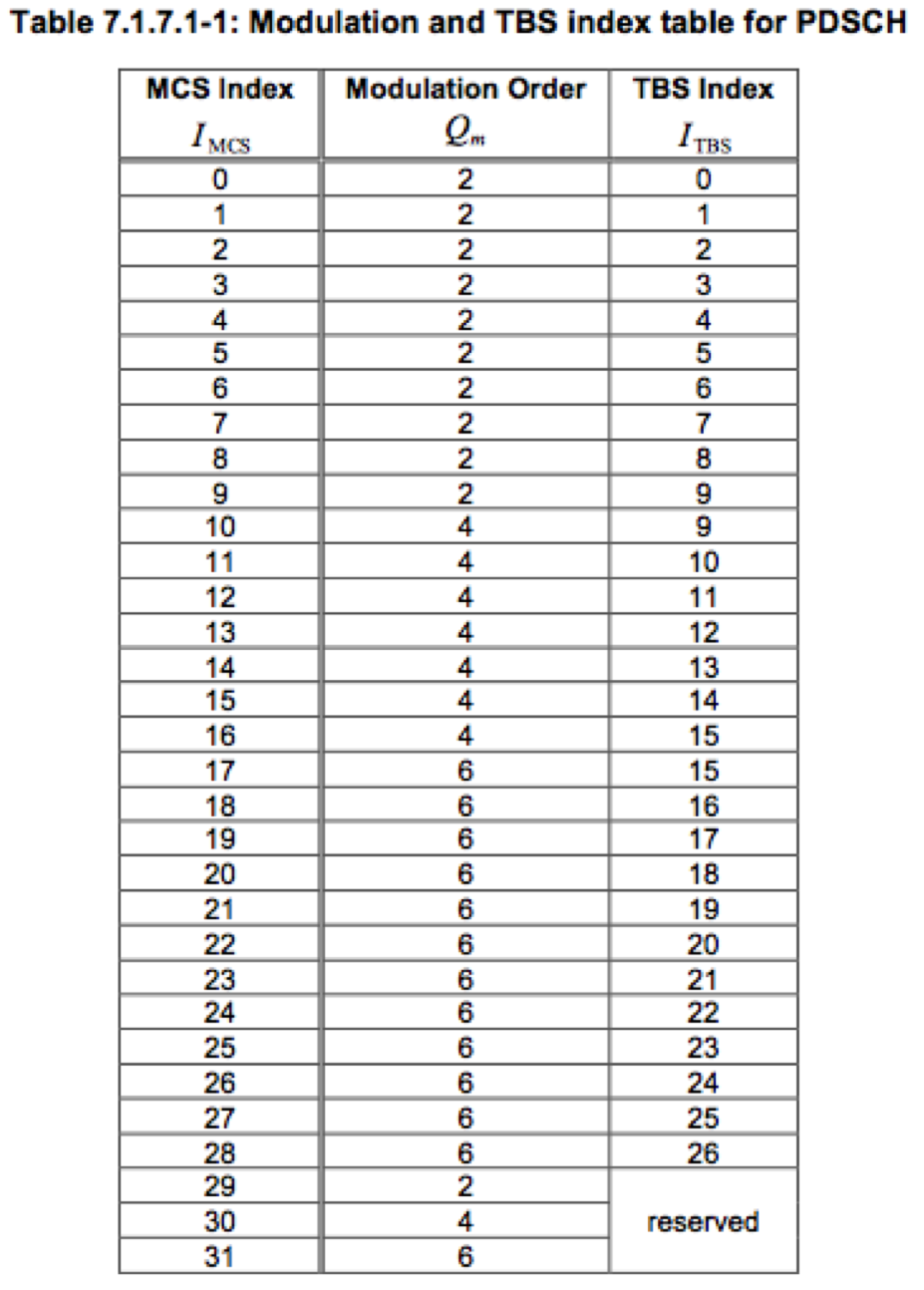Transport Block size and code rate

+1 vote
1,137 views

Two queries -
1. How transport block size is calculated in LTE?
2. Any tutorial on code rate ie what is the significance and how it is computed?posted Aug 16, 2013

1. Transport block size
It depends on the MCS (modulation and coding scheme) and the number of resource blocks assigned to the UE. We have to refer to the Table 7.1.7.1-1 and Table 7.1.7.2.1-1 from 3GPP 36.213Lets assume that eNB assigns MCS index 20 and 2 resource blocks (RBs) on the basis of CQI and other information for downlink transmission on PDSCH. Now the value of TBS index is 18 as seen in Table 7.1.7.1-1

After knowing the value of TBS index we need to refer to the Table 7.1.7.2.1-1 to find the accurate size of transport block (Only portion of the table is shown here while for the complete range of values refer to 3gpp document 36.213 http://www.quintillion.co.jp/3GPP/Specs/36213-920.pdf)Now from the Table 7.1.7.2.1-1 the value of Transport block size is 776 bits for ITBS = 18 and NPRB=2

2 Code Rate
In simple words, code rate can be defined as how effectively data can be transmitted in 1ms transport block or in other words, it is the ratio of actual amount of bits transmitted to the maximum amount of bits that could be transmitted in one transport block

code rate = (TBS + CRC) / (RE x Bits per RE)

where
TBS = Transport block size as we calculated from Table 7.1.7.2.1-1
CRC = Cyclic redundancy check i.e. Number of bits appended for error detection
RE = Resource elements assigned to PDSCH or PUSCH
Bits per RE = Modulation scheme used

While we know the values of TBS, CRC and bits per RE (modulation order), it is not easy to calculate the exact amount of RE used for PDSCH or PUSCH since some of the REs are also used by control channels like PDCCH, PHICH etc

In our case, lets assume that 10% of RE's are assigned for control channels then

TBS = 776
CRC = 24
RE = 2 (RB) x 12 (subcarriers) x 7 (assuming 7 ofdm symbols) x 2 (slots per subframe) x 0.9 (10% assumption as above) = 302 REs
Bits per RE = 6 (Modulation order from table 7.1.7.1-1)

So

code rate = (776 + 24) / (302 * 6 ) = 0.4answer Aug 17, 2013
Similar Questions

In LTE, the transport block size is a mapping of the CQI, MCS and the allocated PRB and does not depend on the CFI. So for the same TBSize (which means same CQI, MCS, allocated PRB), if the CFI is changed (as in case of dynamic CFI) what will happen? is the code rate will be increased or decreased?

In unicast mode, MCS is very dynamic in nature and depends on feedback from UE while in multicast or broadcast there is no feedback from UE then how a particular MCS index is chosen ? And is there any possibility when MCS for an already configured MBSFN can be updated ?

+1 vote

I would like to understand the relation between the TB size calculation in the TS36.213 document and in the Vienna Simulator https://www.nt.tuwien.ac.at/research/mobile-communications/vienna-lte-a-simulators . I’m going to make an example so maybe you can help me to see this relation:

Method 1 (from the TS36.213)

Calculation Procedure for downlink(PDSCH) is as follows :
i) refer to TS36.213 Table 7.1.7.1-1
ii) get I_TBS for using MCS value (Let’s assume MCS is 1. in this case, I_TBS is 1 )
iii) refer to TS36.213 Table7.1.7.2.1
iv) go to column header indicating the number of RB (Let’s assume that RB is 50)
v) go to row header ‘1’ which is I_TBS
vi) we would get 1800 (if the number of RB is 50 and I_TBS is 9)
vii) (This is Transport Block Size per 1 ms for one Antenna)

And this is method 2 (in the simulator):
i) This is the formula:
TB_size_bits = max(8*round(1/8*(the_RB_grid.sym_per_RB_nosync .* num_assigned_RB .* modulation_order .* coding_rate * 2))-24,0);
ii) if the_RB_grid.sym_per_RB_nosyn= 80
iii) num_assigned_RB=50
iv) modulation_order = 2
v) coding_rate=0.0762
vi) That gives TB_size_bits = 11952 bits

Do you know how I can go from one method to another or if there is any relation?

+1 vote

How can we calculate the transport block size given the MCS index and the number of used PRBs as defined in the 3GPP table 36.213.